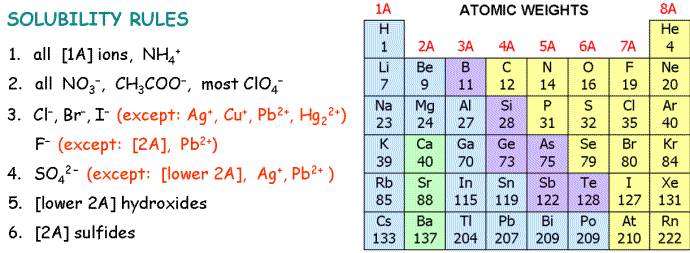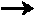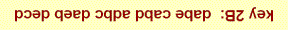## Energy:          1 cal = 4.184 JSelect the best single answer for each question:

1.  Which is a strong electrolyte?

1.  HCl                2.   KOH                3.  HNO2

 A) 1 only B) 2 only C) 3 only D) 1 and 2 only E) 1, 2 and 3 only

2.  The total moles of ions released when 3 moles of Al2(SO4)3 dissolve completely in water is

 A) 15 B) 12 C) 9 D) 18 E) 21

3.  When HNO3 reacts with KOH in aqueous solution the net ionic equation is

 A) K+(aq) + NO3- (aq)KNO3 (s) B) OH-(aq) + H+(aq)H2O(l) C) H+(aq) + KOH(aq)K+(aq) + H2O(l) D) OH-(aq) + HNO3(aq)H2O(l) + NO3- (aq)

4.   It takes 10,600 kJ each day to maintain a body weight of 80 kg (about 175 lb).
How many kilocalories is this each day?
 A) 1275 B) 4435 C) 2190 D) 3548 E) 2533

5.   Which of these compounds is most soluble in water?

 A) CuS B) PbSO4 C) ZnCl2 D) Ag2CO3

6.   Oxidation-reduction reactions involve transfer of

 A) electrons from the reducing agent to the oxidizing agent B) protons from the oxidizing agent to the reducing agent C) electrons from the oxidizing agent to the reducing agent D) protons from the reducing agent to the oxidizing agent

7. How many mL of 0.200 M NaOH neutralize 50.0 mL of 0.760 M HNO3 ?

 A) 124 B) 190 C) 232 D) 168 E) 215

8. The oxidation number of vanadium in HV6O173-  is

 A) 9 B) 8 C) 4 D) 5 E) 6

9.   Which is NOT an oxidation-reduction reaction?

 A) 4 AgNO3 +  SnCl44 AgCl +  Sn(NO3)4 B) S8 + 24 F28 SF6 C) ClO- + NO2-NO3- + Cl- D) 2 Cr + 3 Sn2+2 Cr3+ + 3 Sn

10.   Find the oxidizing agent in the equation

6 Ag+ + 3 S + 2 NO + 4 H2O3 Ag2S + 8 H+ + 2 NO3-

 A) NO B) H2O C) Ag+ D) S

11.   Use the data to find the enthalpy change for the reaction Br + H2HBr + H

H2H + H ,        DH = 436 kJ
Br2Br + Br,       DH = 193 kJ
HBrH + Br,        DH = 366 kJ

 A) -243 kJ B) 70 kJ C) 173 kJ D) 243 kJ E) -173 kJ

12.   A chemical process at constant pressure transfers 200 kJ of heat to the surroundings.
The enthalpy change of the system is best described as:

 A) DH =  200 kJ (endothermic) B) DH =  200 kJ (exothermic) C) DH = -200 kJ (exothermic) D) DH = -200 kJ (endothermic)

13.   How much energy (J) is required to heat 151 kg of water (about 40 gal in a home water heater)

from 18.0°C to 50.0°C?   The heat capacity of water is 4.184 J/g-°C.

 A) 2.65 ´ 107 J B) 1.52 ´ 107 J C) 9.96 ´ 106 J D) 2.02 ´ 107 J E) 1.52 ´ 104 J

14. Which of these has the highest oxidation number for sulfur?

 A) Na2S2O8 B) H2S C) SO2 D) S8

15.   How many mL of 1.00 M HCl react with 0.0350 moles of calcium hydroxide by

Ca(OH)2 + 2 HClCaCl2  + 2 H2O

 A) 50.0 B) 35.0 C) 60.0 D) 25.0 E) 70.0

16. Use the heats of formation to find DH° for the reaction

 N2O(g) + 3H2(g)N2H4(l)  + H2O(g) DH°f A) -153 kJ N2O(g) 82 kJ B) -273 kJ N2H4(l) 51 kJ C) -133 kJ H2O(l) -286 kJ D) -204 kJ H2O(g) -242 kJ E) -317 kJ

17.  Mixing solutions of barium hydroxide and magnesium sulfate yields

 A) aqueous barium sulfate and solid magnesium hydroxide B) aqueous barium sulfate and aqueous magnesium hydroxide C) solid barium sulfate and aqueous magnesium hydroxide D) solid barium sulfate and solid magnesium hydroxide

18. Find the number of electrons transferred in the balanced oxidation-reduction reaction

2 C6H6 + 15 O212 CO2  +  6 H2O

 This type of problem will NOT be covered on the exam. A) 24 B) 40 C) 48 D) 36 E) 60

19.   56.5 mL of  0.100M NaOH solution neutralizes 260 mg of an unknown acid HA.
Which of the following acids has a molecular weight closest to the unknown?

 A) HOCl B) HCN C) HCOOH D) HF E) CH3COOH

20.    The standard heat of formation of H2O(g) is the enthalpy change for the reaction

 A) 2H(g) + O(g)H2O(g) B) 2H2(g) + O2(g)2H2O(g) C) H2O(g)2H(g) + O(g) D) H2(g) + 0.5 O2(g)H2O(g) E) H2O(l)H2O(g)